For those wishing to follow along with the R-based demo in class, click here for the companion R script for this lecture.

Bayesian analysis is likelihood-based, and follows naturally from our previous discussions. The difference is that we are no longer interested in the maximum likelhood estimate (MLE) and the properties of maximum likelhood estimators. Instead, we are now interested in using the likelihood function to update our degree of belief in all possible parameter values. Effectively, we want a joint probability distribution (called the joint posterior) that reflects our degree of belief in all possible parameter values (potentially across a many-dimensional parameter space) after we have observed some relevant data.

### Play with binomial/beta (conjugate prior)

Let’s imagine we know N (N, the number of independent trials, is fixed), but we want to estimate p. For now, we will assume we have no prior information about p, such that any value of p is equally likely.

#### Set the prior

To set the prior, let’s assume p follows a uniform distribution between 0 and 1:

###################
### Bayesian analysis example using Binomial distribution

######
# first visualize the "prior" for the probability *p* as a uniform distribution

curve(dunif(x),ylim=c(0,2),col="red", ylab = "probability", xlab="parameter \"p\"")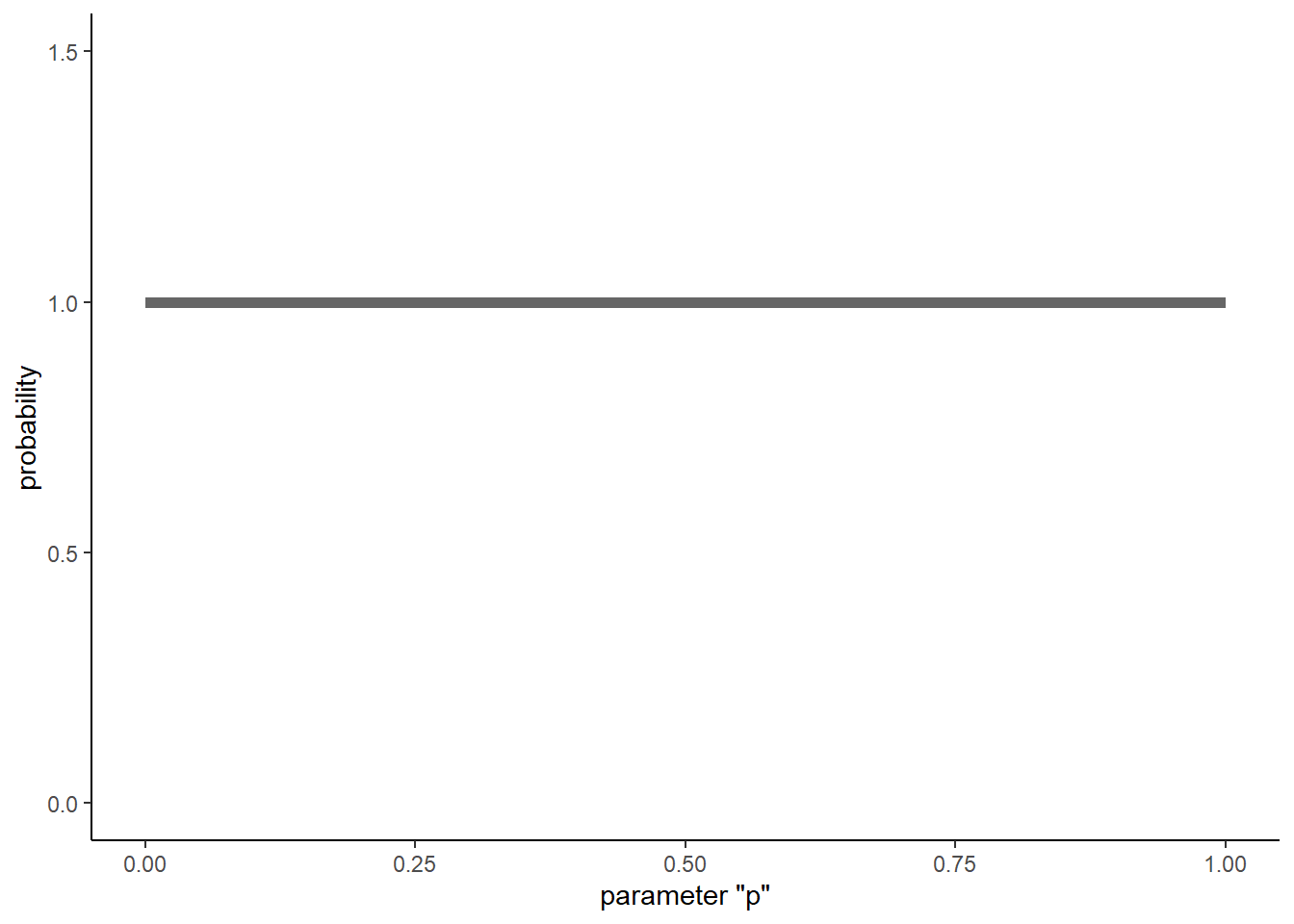#hist(runif(10000),freq=F,ylim=c(0,2),col="red")

An alternative way to specify this uniform (flat) prior is to use the beta distribution, with both parameters of the distribution (shape1 and shape2) set to 1

#############
# Alternative prior: beta distribution (conjugate prior)

curve(dbeta(x,1,1),ylim=c(0,2),col="red",ylab = "probability", xlab="parameter \"p\"")

#hist(rbeta(10000,1,1),freq=F,ylim=c(0,2),col="red")   # histogram of random numbers from a flat beta distribution

### Conjugate prior

Why choose the beta distribution here? The answer is that the beta distribution is the conjugate prior for the p parameter in the binomial distribution. This makes Bayesian estimation easy and straightforward, as we will see!

#### Definition: conjugate prior

A conjugate prior is a distribution for a parameter or set of parameters that matches the data-generating model: that is, the prior has the same general form as the likelihood function. One consequence of this is that prior and the posterior distribution can be represented with the same type of distribution (e.g., gamma, beta, normal). We will come back to this!

### Worked example

Let’s work through an example. Take the same frog-call survey we have imagined before (a simple problem with binomial data). Recall that a particular site is occupied. After visiting the site 10 times, we detected the frog (heard its call) 3 times out of 10 total visits. We are interested in determining the detection probability.

We know the likelihood of the data across parameter space. So now we have both a prior distribution for our parameter of interest, as well as a likelihood surface.

#############
# frog call example: imagine we detected the frog in 3 of 10 visits to a known-occupied wetland

######
# visualize the data likelihood alongside the prior probability

#   # recall that likelhood surface is not a probability distribution (thus, the 2 y axes)

data = 3
param.space <- seq(0,1,by=0.001)
likelihood <- dbinom(data,size=10,prob=param.space)
par(mai=c(1,1,0,1))
curve(dbeta(x,1,1),ylim=c(0,2),col="blue",lty=2,ylab="Probability density (prior)",xlab="parameter (p)")
points(param.space,likelihood*5,type="l",col="red",lwd=2,lty=2)
axis(4,at=seq(0,2,by=0.4),labels = seq(0,0.5,by=.1))
mtext("Likelihood (relative plausibility)", side=4, col="red",line=3)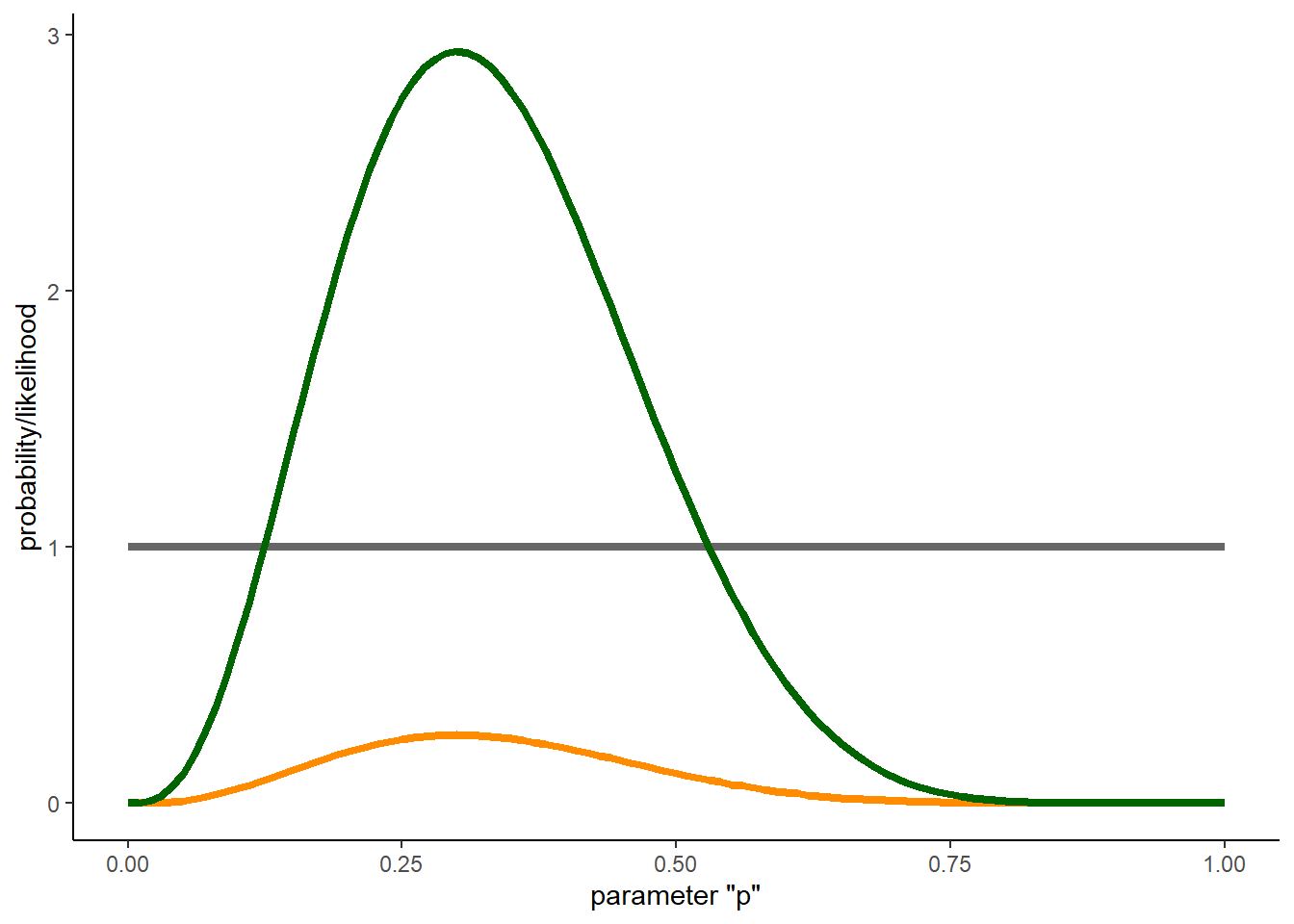Recall that the likelihood curve is NOT a probability distribution. It does not sum to 1! In Bayesian analyses, we translate the likelihood to a probability distribution using Bayes rule!!

$$Prob(Model|Data) = \frac{Prob(Data|Model)\cdot Prob(Model))}{Prob(Data)}$$

The likelihood is just the $$Prob(Data|Model)$$ term…

What is the unconditional probability of the data ($$Prob(Data)$$, also known as the “evidence”)? Well, it’s really the sum of the probability of the data across all possible (mututally exclusive) parameter values (parameter space), weighted by the prior. This evaluates to a single number, and can be seen as a normalizing constant that is used to convert the numerator of Bayes rule into a probability distribution.

As always (in this class), let’s do it first by brute force!!

###########
# Brute-force Bayes

####
# prior across parameter space

prior <- dbeta(param.space,shape1=1,shape2=1)    # flat prior
#prior

######
## Numerator for Bayes rule: weight the data likelihood by the prior

weighted.likelihood <- likelihood*prior      # Numerator for Bayes rule

######
## Denominator for Bayes rule: compute normalization constant

normalization.constant <- sum(weighted.likelihood)   # "evidence" is a single constant number: the sum of the weighted likelihoods!

######
## Posterior!! (numerator/denominator)

posterior <- weighted.likelihood/normalization.constant   # this is Bayes' rule!

#######
## Plot it out!

par(mai=c(1,1,0,1))
plot(param.space,prior,ylim=c(0,5),type="l",lwd=1,lty=2,col="blue",ylab="Probability Density",xlab="param.space")
points(param.space,posterior*length(param.space),type="l",col="blue",lwd=2,lty=1)  # convert posterior to probability density
points(param.space,likelihood*5,type="l",col="red",lwd=1,lty=2)
axis(4,at=seq(0,2,by=0.4),labels = seq(0,0.5,by=.1))
mtext("Likelihood", side=4, col="red",line=3)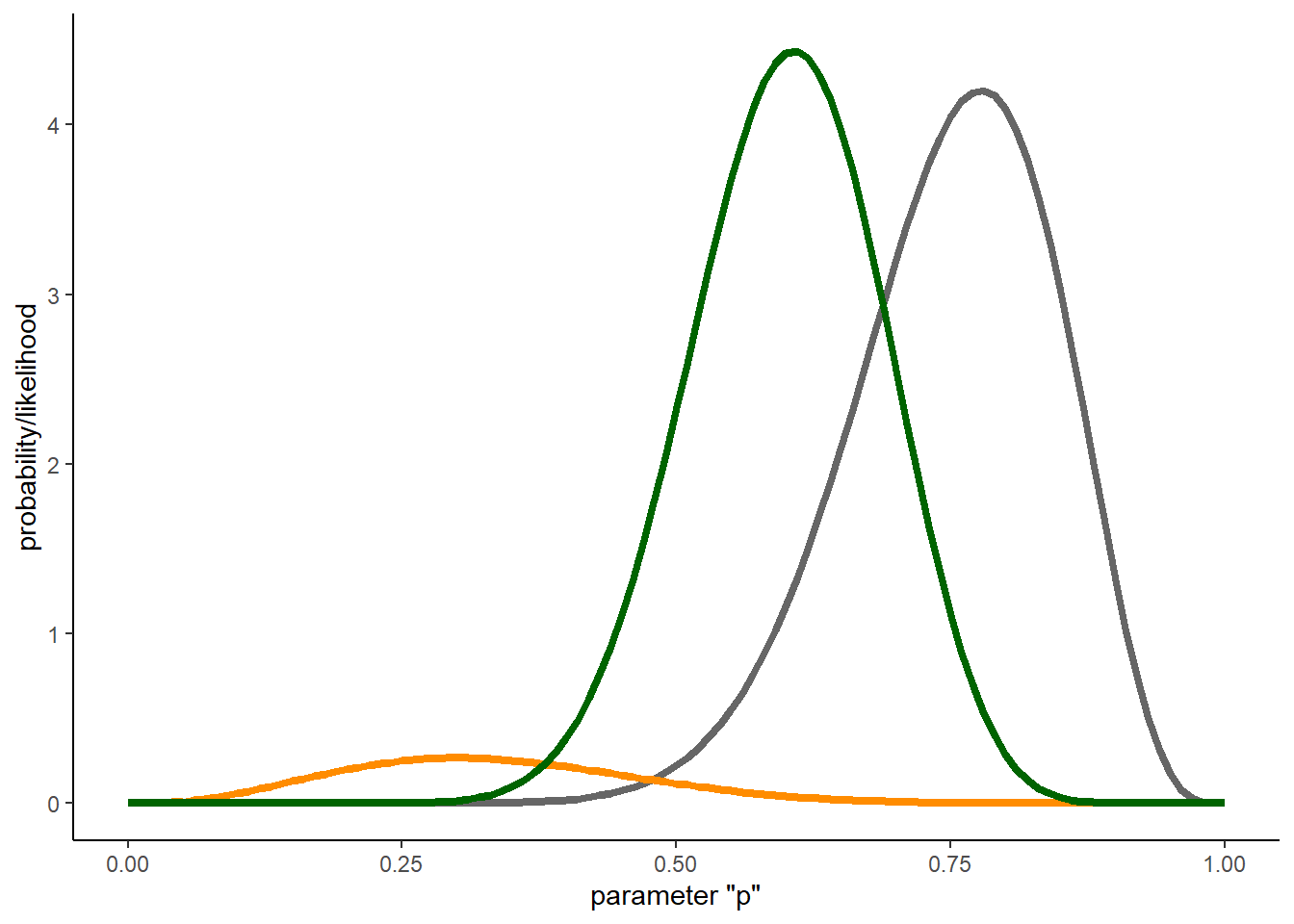Notice that the shape of the posterior looks a lot like the shape of the likelhood surface. What this says to us is that the prior has been overwhelmed by the information content of the data (as summarized by the likelihood surface).

Again: recall that the likelhood surface is NOT a probability distribution- it is a way to assess the relative plausibility of the data across parameter space. The prior and the posterior are probability distributions, and necessarily integrate to 1.

#############
# Try an informative prior!

prior <- dbeta(param.space,shape1=15,shape2=5)
#prior

## weight the data likelihood by the prior

weighted.likelihood <- likelihood*prior

## compute normalization constant

normalization.constant <- sum(weighted.likelihood)

## Posterior!!

posterior <- weighted.likelihood/normalization.constant

## Plot it out!
par(mai=c(1,1,0,1))
plot(param.space,prior,ylim=c(0,5),type="l",lwd=1,lty=2,col="blue",ylab="Probability Density",xlab="param.space")
points(param.space,posterior*length(param.space),type="l",col="blue",lwd=2,lty=1)
points(param.space,likelihood*5,type="l",col="red",lwd=1,lty=2)
axis(4,at=seq(0,2,by=0.4),labels = seq(0,0.5,by=.1))
mtext("Likelihood", side=4, col="red",line=3)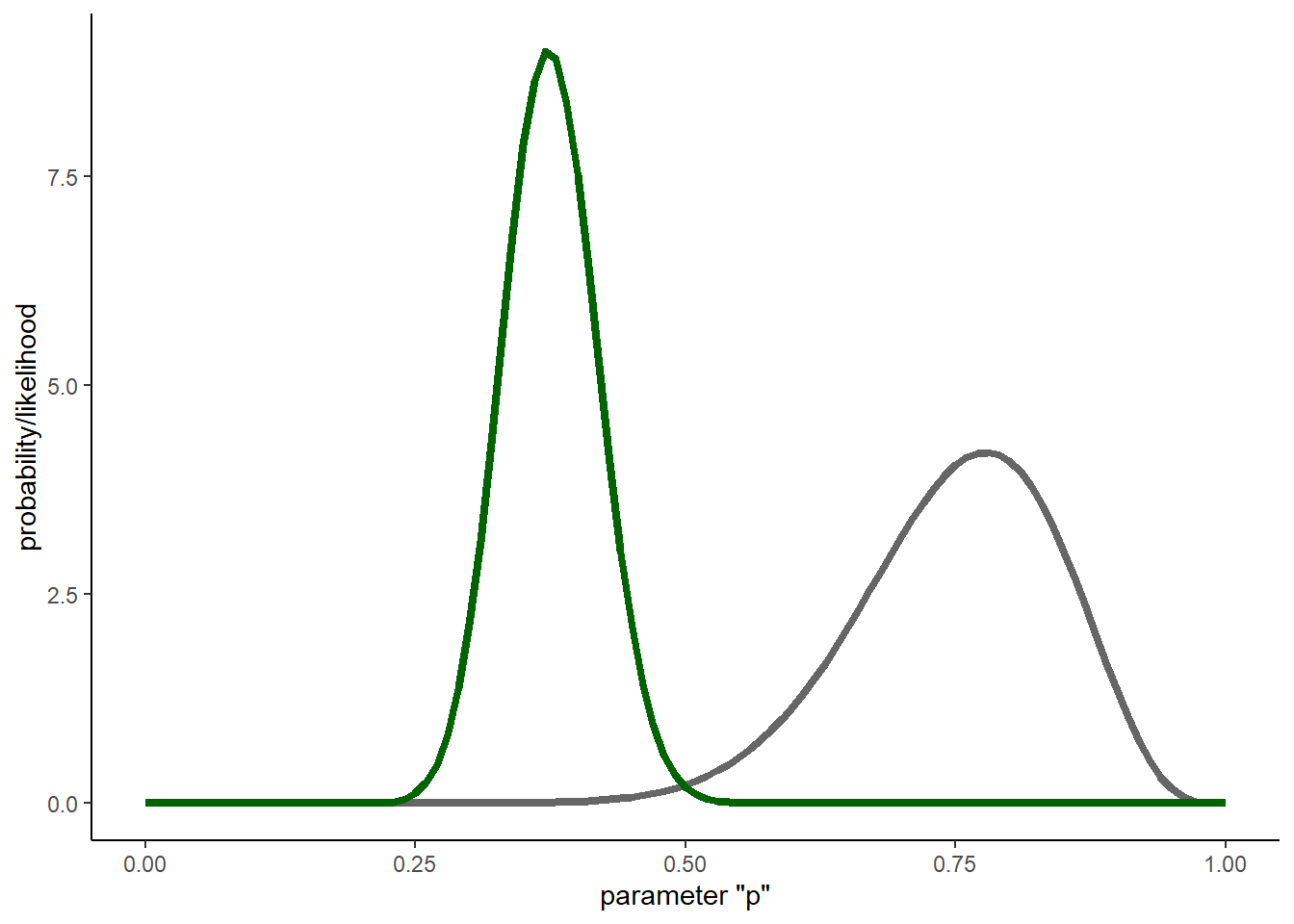What does this tell us?

What about if we have more data? Let’s imagine we visited 10 occupied ponds and observed the following data:

3, 1, 6, 2, 3, 2, 6, 1, 3, 3

############
# Collect more data and try again...

moredata <- c(3, 1, 6, 2, 3, 2, 6, 1, 3, 3)

## prior
prior <- dbeta(param.space,shape1=15,shape2=5)

## likelihood
likelihood <- sapply(param.space,function(t) prod(dbinom(moredata,size=10,prob=t)))

## weight the data likelihood by the prior
weighted.likelihood <- likelihood*prior

## compute normalization constant

normalization.constant <- sum(weighted.likelihood)

## Posterior!!

posterior <- weighted.likelihood/normalization.constant

## Plot it out!
par(mai=c(1,1,0,1))
plot(param.space,prior,ylim=c(0,10),type="l",lwd=1,lty=2,col="blue",ylab="Probability Density",xlab="param.space")
points(param.space,posterior*length(param.space),type="l",col="blue",lwd=2,lty=1)
points(param.space,likelihood*1e9,type="l",col="red",lwd=1,lty=2)
axis(4,at=seq(0,6,by=1),labels = seq(0,6e-9,by=1e-9))
mtext("Likelihood", side=4, col="red",line=3)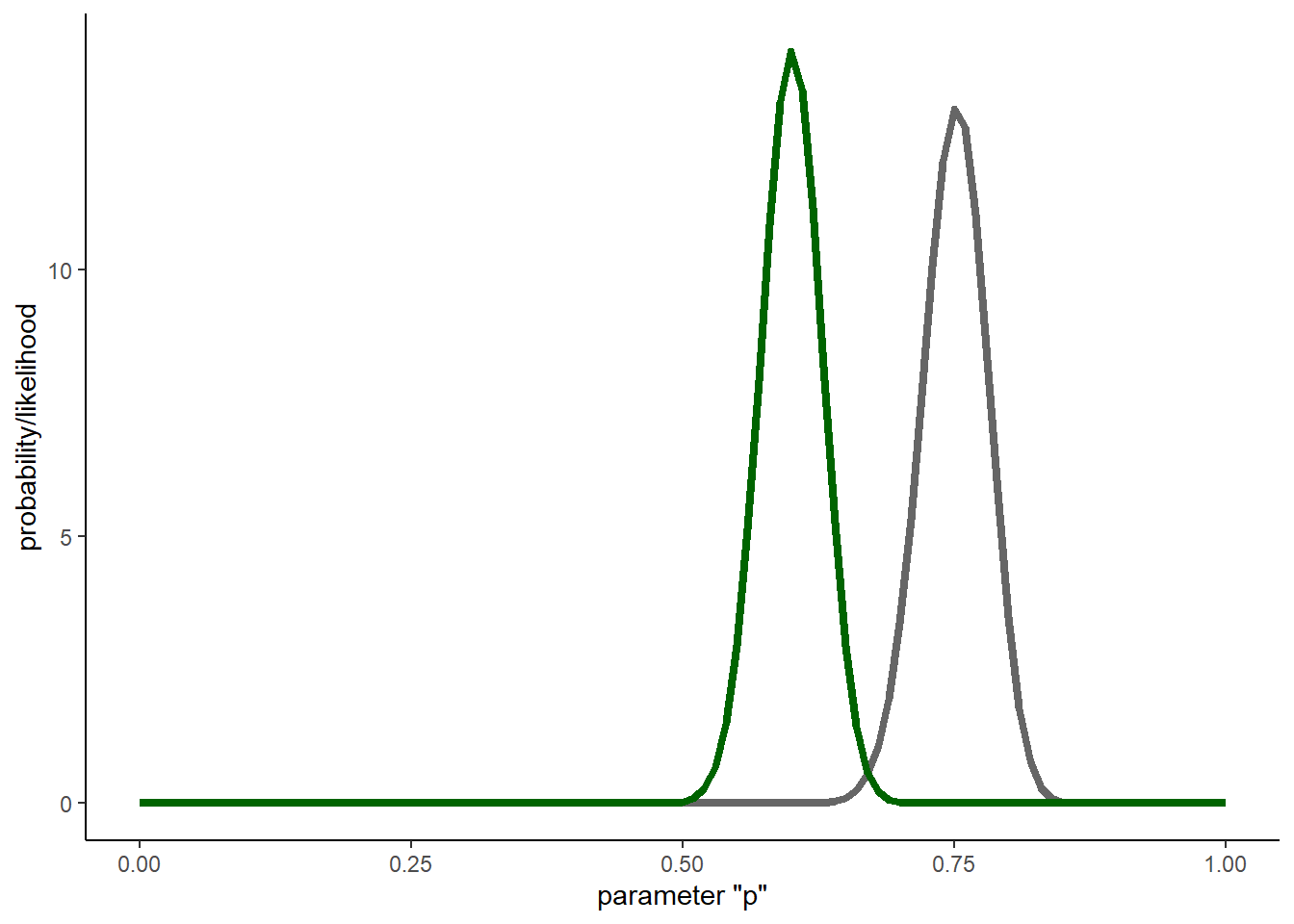#######
# Try a very informative prior!

likelihood <- dbinom(data,size=10,prob=param.space)

What about a super informative prior??

prior <- dbeta(param.space,shape1=150,shape2=50)
#prior

## weight the data likelihood by the prior

weighted.likelihood <- likelihood*prior

## compute normalization constant

normalization.constant <- sum(weighted.likelihood)

## Posterior!!

posterior <- weighted.likelihood/normalization.constant

## Plot it out!
par(mai=c(1,1,0,1))
plot(param.space,prior,ylim=c(0,15),type="l",lwd=1,lty=2,col="blue",ylab="Probability Density",xlab="param.space")
points(param.space,posterior*length(param.space),type="l",col="blue",lwd=2,lty=1)
points(param.space,likelihood*5,type="l",col="red",lwd=1,lty=2)
axis(4,at=seq(0,2,by=0.4),labels = seq(0,0.5,by=.1))
mtext("Likelihood", side=4, col="red",line=3)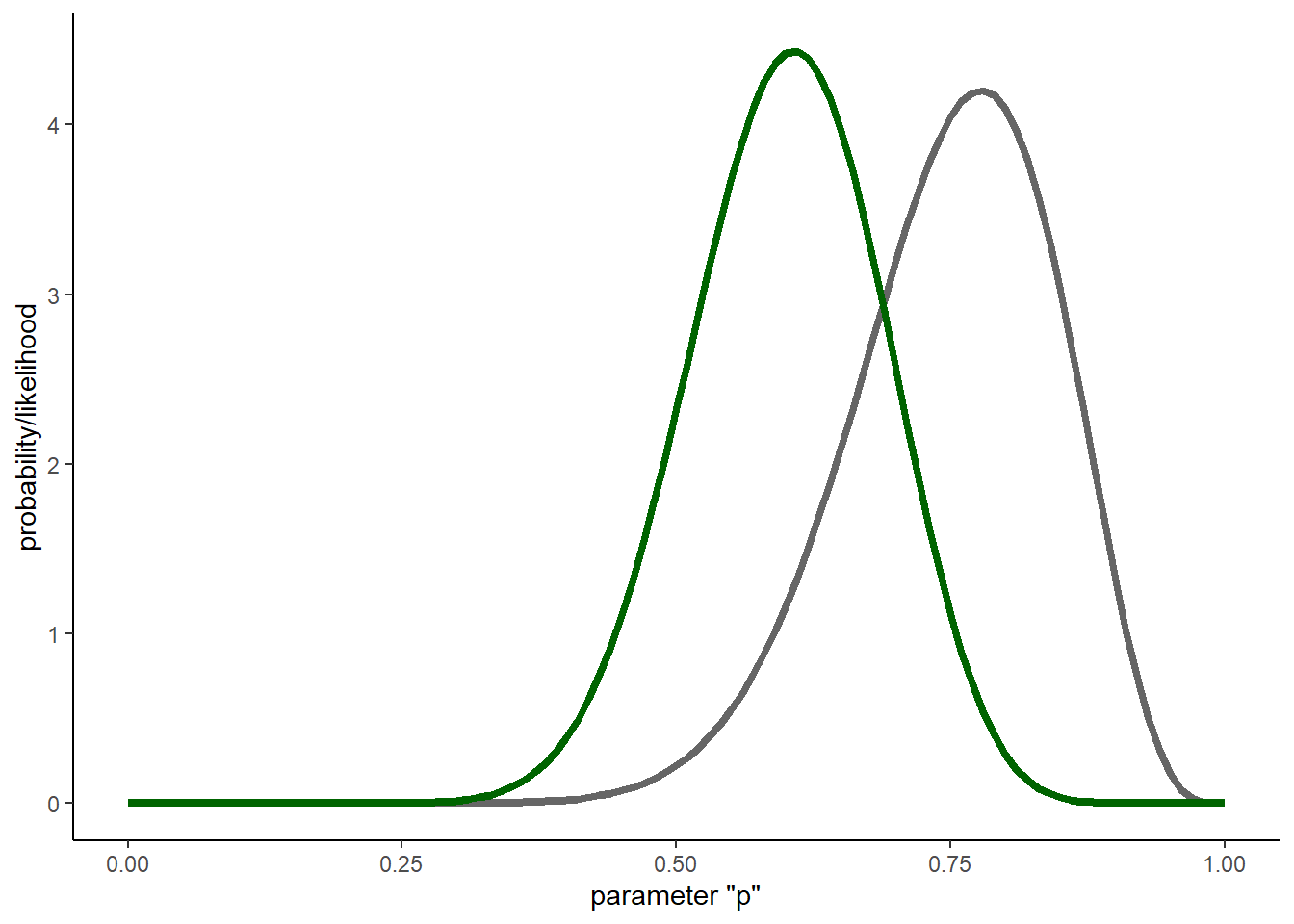Okay, now let’s do it the more mathematically elegant way! When we work with a conjugate prior, the updating process is easy. The posterior distribution for the p term in the above example can be computed by:

$$Beta(shape1=prior+k,shape2=prior+(N-k))$$

Let’s do the same thing, now using the conjugate prior method…

###############
# Do it again- this time with conjugate priors...

### PRIOR

prior_beta <- c(shape1=1,shape2=1)
curve(dbeta(x,prior_beta['shape1'],prior_beta['shape2']),ylim=c(0,5),ylab="Prob Density",col="blue",lwd=1,lty=2,xlab="param.space")

### POSTERIOR

curve(dbeta(x,prior_beta['shape1']+data,prior_beta['shape2']+(10-data)),ylim=c(0,4),ylab="Prob Density",col="blue",lwd=2,lty=1,xlab="param.space",add=T)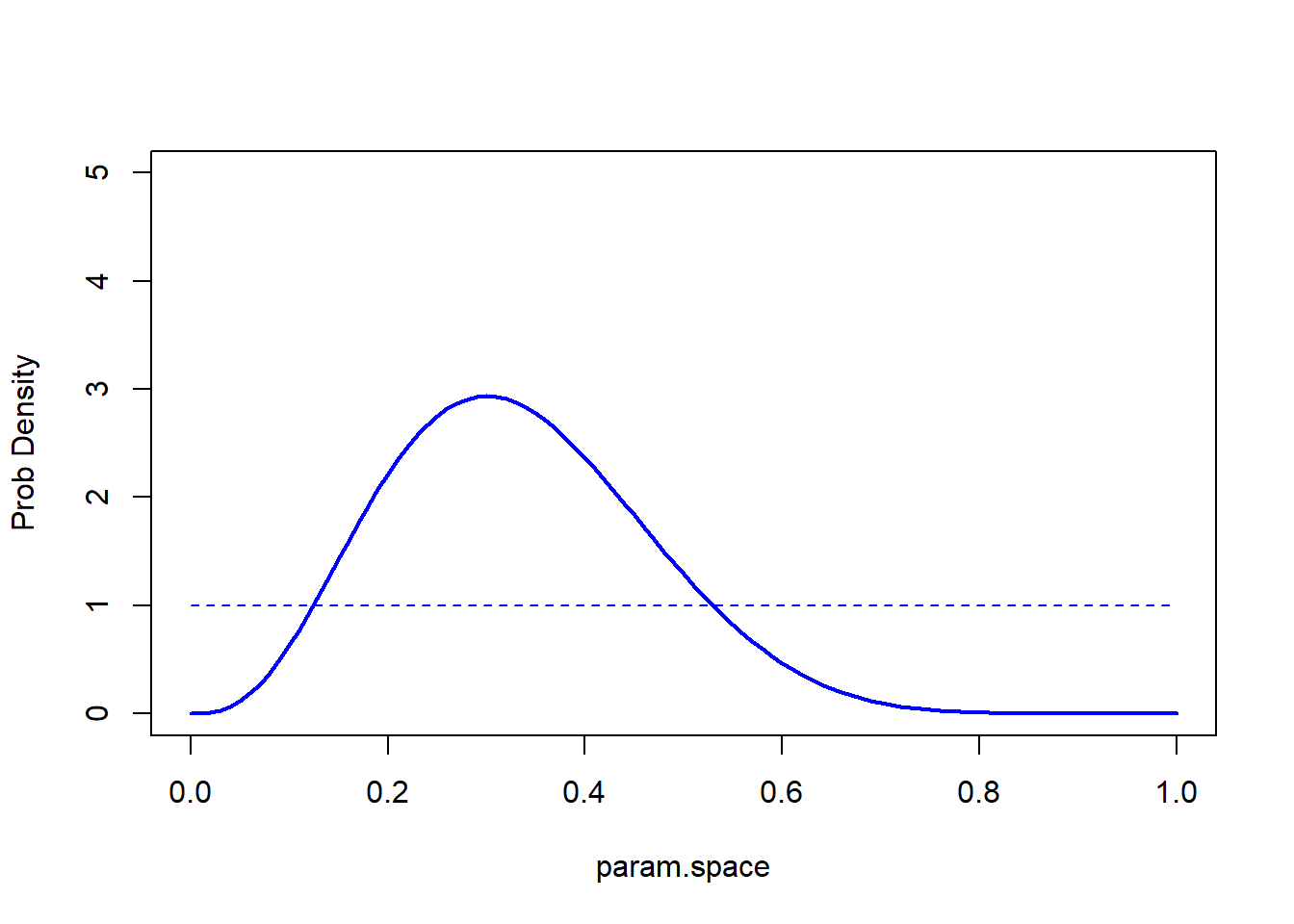And again, this time with an informative prior!

#########
# With informative prior...

### PRIOR
prior_beta <- c(shape1=15,shape2=5)
curve(dbeta(x,prior_beta['shape1'],prior_beta['shape2']),ylim=c(0,5),ylab="Prob Density",col="blue",lwd=1,lty=2,xlab="param.space")

### POSTERIOR

curve(dbeta(x,prior_beta['shape1']+data,prior_beta['shape2']+(10-data)),ylim=c(0,4),ylab="Prob Density",col="blue",lwd=2,lty=1,xlab="param.space",add=T)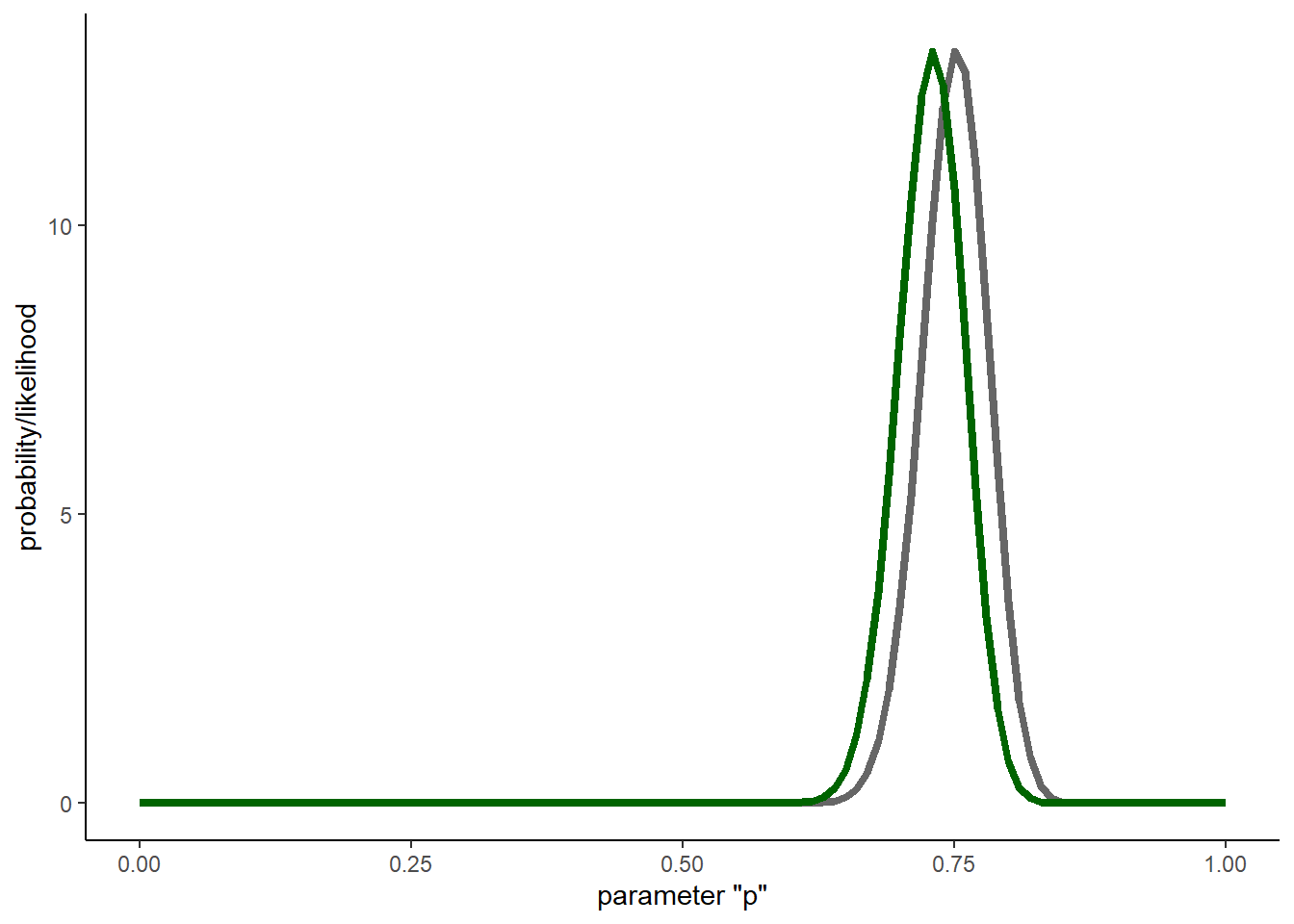graphics.off()

And the super informative prior??

########
# And with super informative prior...

### PRIOR
prior_beta <- c(shape1=150,shape2=50)
curve(dbeta(x,prior_beta['shape1'],prior_beta['shape2']),ylim=c(0,15),ylab="Prob Density",col="blue",lwd=1,lty=2,xlab="param.space")

### POSTERIOR
curve(dbeta(x,prior_beta['shape1']+data,prior_beta['shape2']+(10-data)),ylim=c(0,15),ylab="Prob Density",col="blue",lwd=2,lty=1,xlab="param.space",add=T)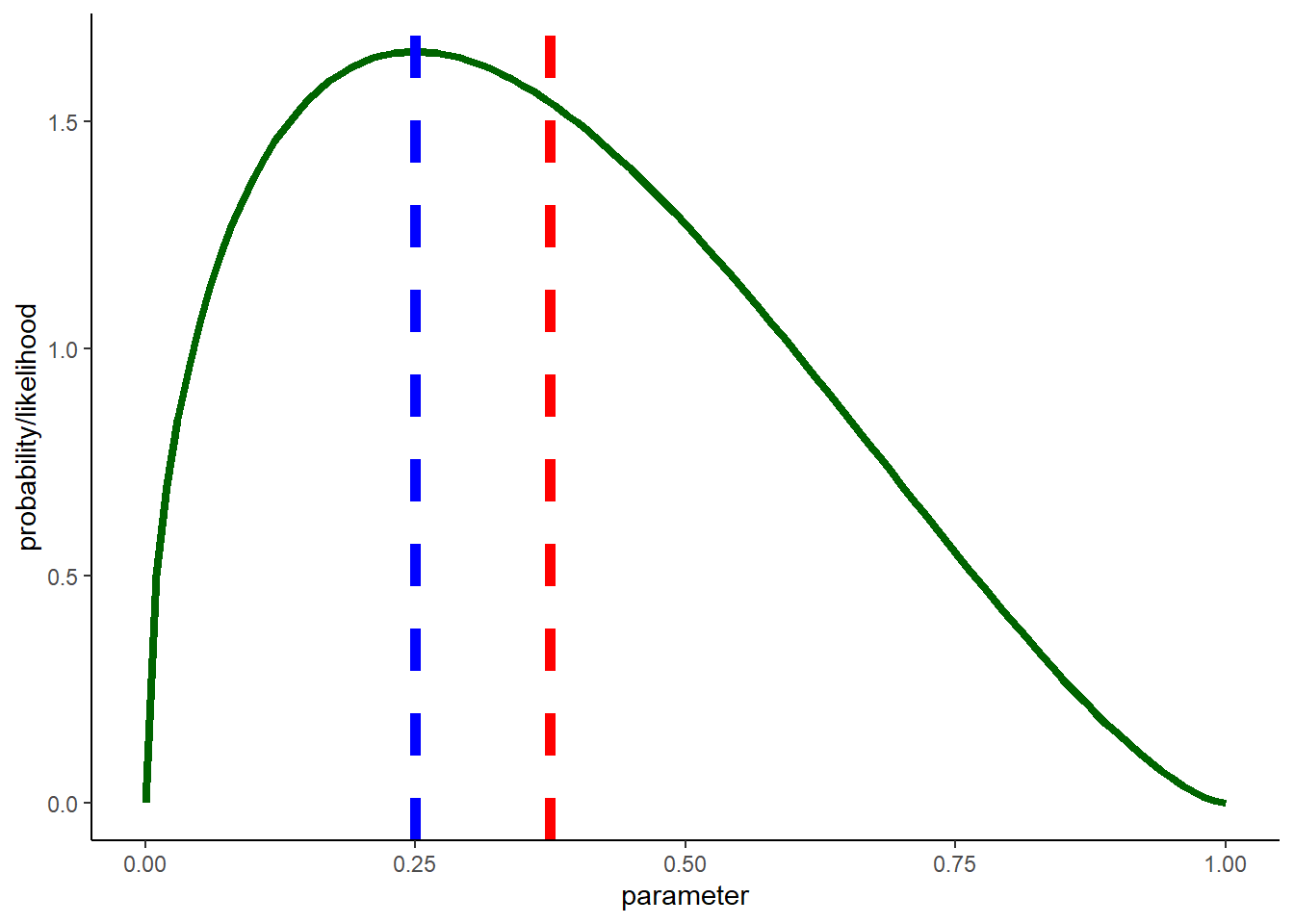### Bayesian point estimate

One of the differences between the MLE and the Bayesian paradigm (although both use likelihood as a way to summarize the information content of the data) is that the point estimate is not usually the maximum (mode) of the posterior distribution (in MLE, we by definition try to find the parameter value that maximizes the likelihood function) but the mean (expected value) or the median of the posterior distribution.

Imagine you had a skewed posterior distribution that looked something like this (this is actually a lognormal distribution):

##########
# Example: bayesian point estimates can differ markedly from MLE

curve(dlnorm(x,4,1),from=0.001,to=200,ylab="prob density")  # use a lognormal distribution for example of skewed posterior dist...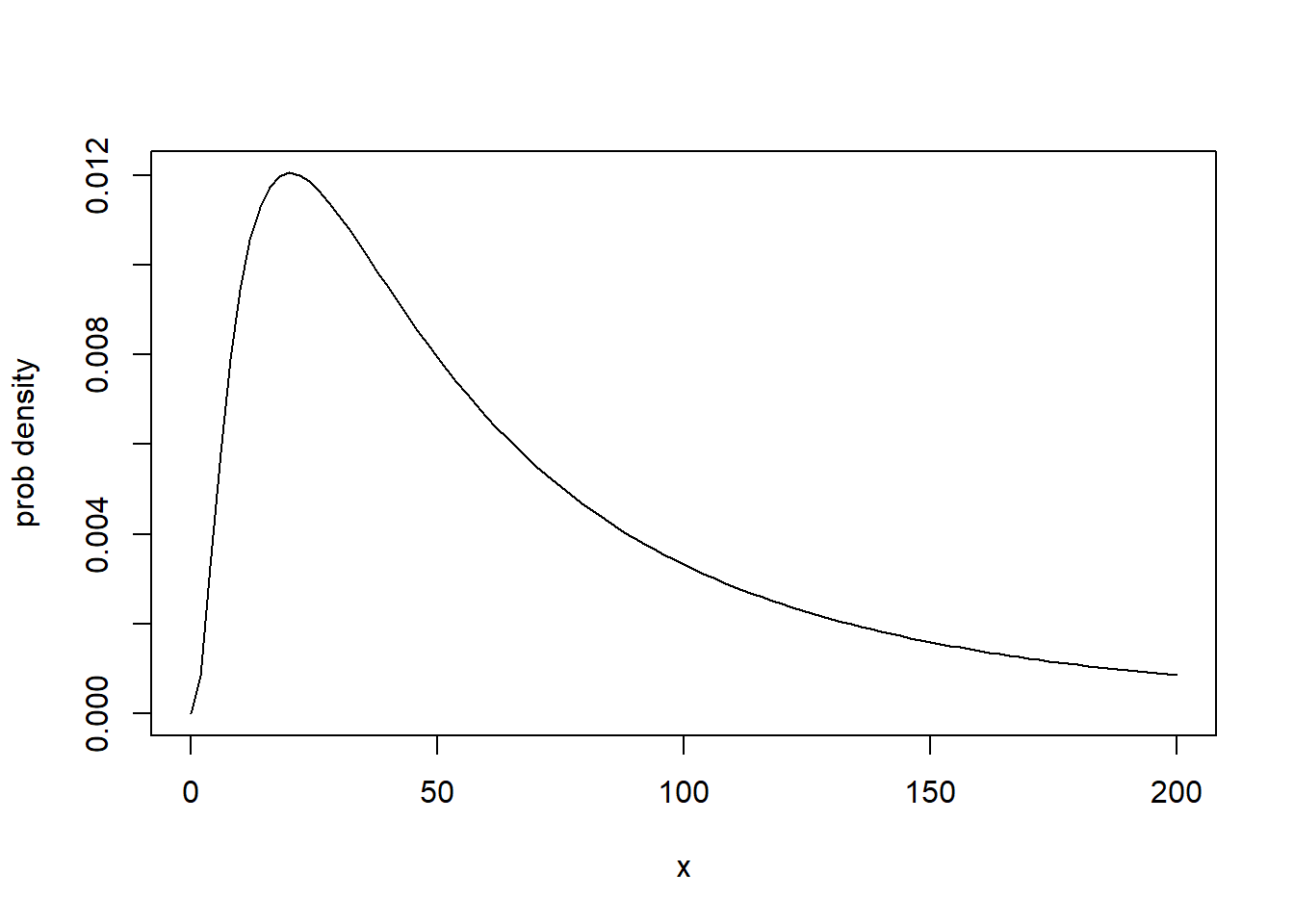Where is the mode? Where is the mean?

##########
# Compute and plot the mean and the mode of the distribution

param.space2 <- seq(0.001,200,length=10000)
skewed.posterior <- dlnorm(param.space2,4,1)
mean <- mean(rlnorm(10000,4,1))
mode <- param.space2[which.max(skewed.posterior)]
plot(param.space2,skewed.posterior,type="l",ylab="prob density")
abline(v=c(mean,mode),col=gray(0.5),lwd=3,lty=2)   # add to plot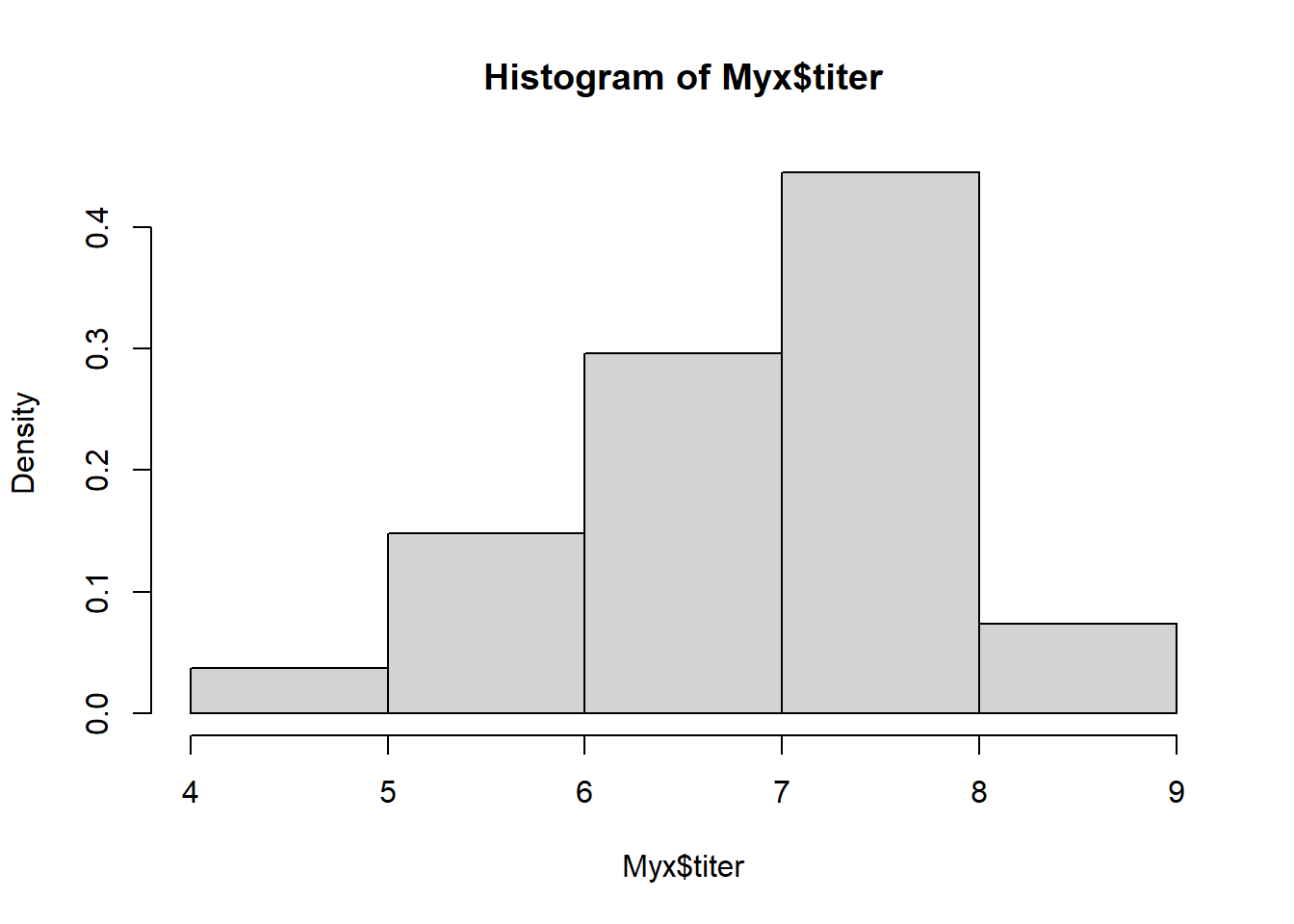What about for the first worked example? Is there a big difference between the mean and the mode?

############
# Do the same for the frog detection example from above

#graphics.off()
### POSTERIOR
posterior <- dbeta(param.space,1+data,1+(10-data))
mean <- mean(rbeta(10000,1+data,1+(10-data)))
mode <- param.space[which.max(posterior)]
plot(param.space,posterior,type="l",col="blue",lwd=2)
abline(v=c(mean,mode),col=gray(0.5),lwd=3,lty=2)   # add to plot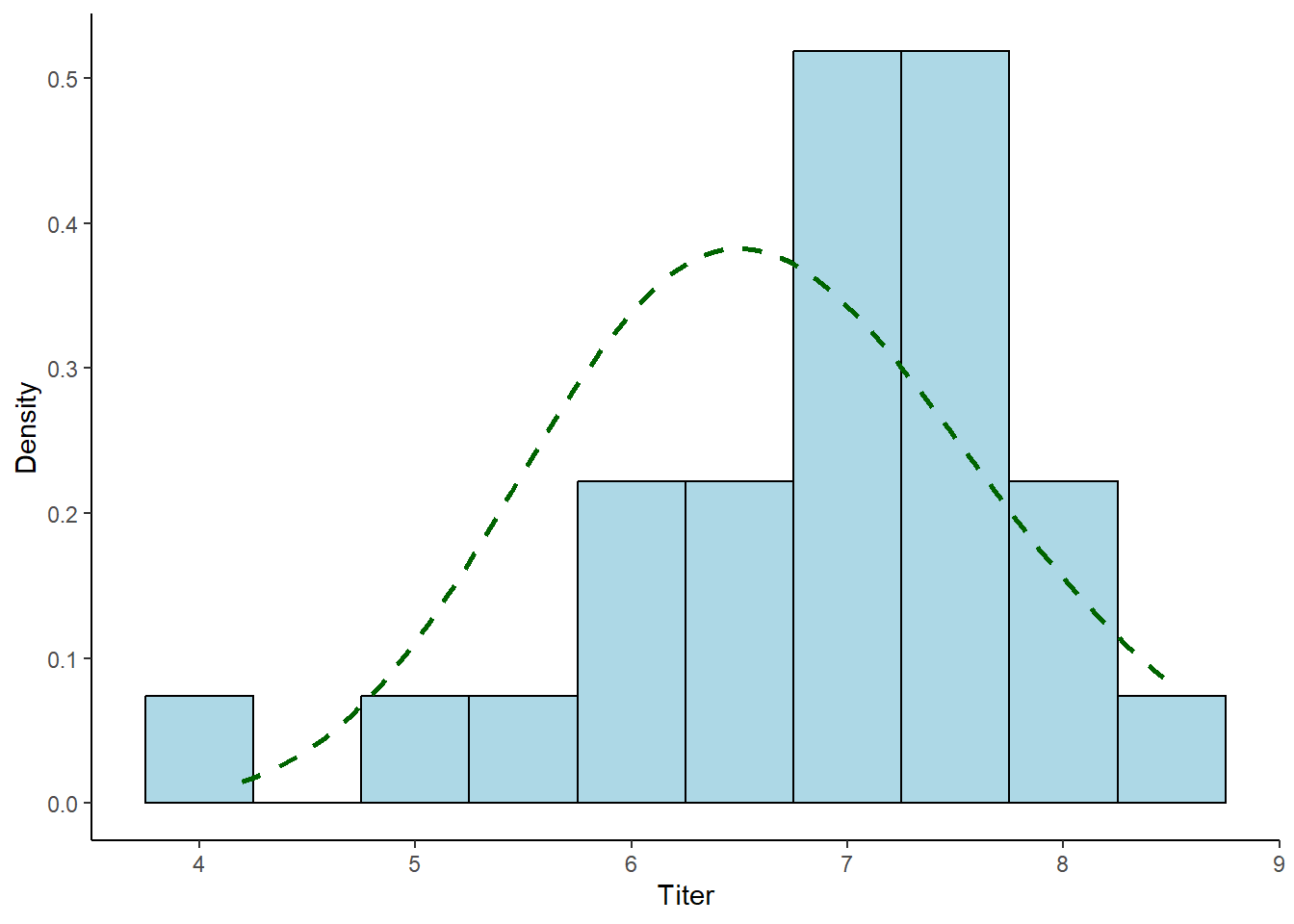#### Thought question

In MLE, we by definition try to find the parameter value that maximizes the likelihood function. Why don’t we use the mean of the likelihood function in Frequentist statistics? In Bayesian analysis, why don’t we use the mode of the posterior distribution?

### Bayesian parameter uncertainty

We often call Bayesian confidence intervals credible intervals to distinguish from their frequentist analog. Bayesian (e.g., 95%) credible intervals can be interpreted in the way you probably have always wanted to interpret frequentist (95%) confidence intervals. It will probably feel satisfying, but a little dirty at the same time (at least at first)!

You are 95% sure that the parameter value is between the lower and upper bound!!!

Why can’t we say this in a frequentist framework?

Let’s try this:

#############
# A Bayesian confidence interval (95% credible interval)

### POSTERIOR

curve(dbeta(x,1+data,1+(10-data)),ylim=c(0,4),ylab="Prob Density",col="blue",lwd=2,lty=1,xlab="param.space")

### CREDIBLE INTERVAL

credible.interval <- qbeta(c(0.025,0.975),1+data,1+(10-data))     # get the credible interval using the quantile method

abline(v=credible.interval,col=gray(0.5),lwd=3,lty=2)   # add to plot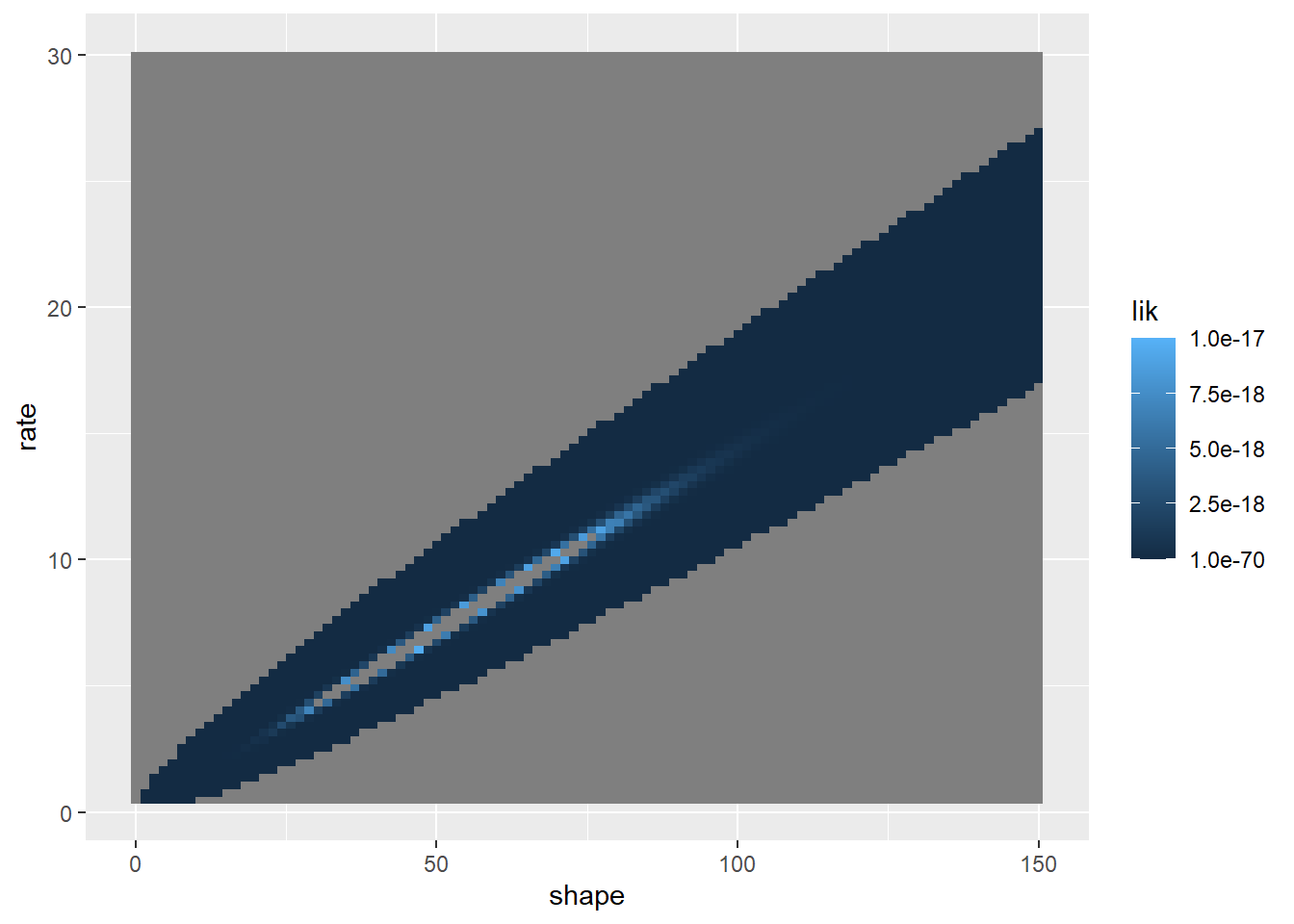#### Highest Posterior Density (HPD) intervals

There are many possible Bayesian credible intervals, even assuming we want a 95% CI. That is, there are many intervals that enclose 95% of the posterior distribution. The Highest Posterior Density interval is the one that is the shortest out of all possible intervals. This is the natural choice for a Bayesian CI since it represents the collection of the most likely values for the paramters - every point within the interval is more plausible than any point outside the interval.

NOTE: the HPD can be discontinuous for multimodal posterior distributions!

### What if there is no nice easy conjugate prior?

One of the reasons Bayesian analysis was less common historically was that there were no mathematically straightforward ways to do the analysis. There still are not BUT we have fast computers and computational algorithms. Basically, we can use various forms of more-or-less brute force computation, along with some mathematical tricks, to complete most Bayesian analyses.

Let’s start with the brutest of brute-force methods (not possible in high-dimensional systems):

### The brute force method

For continuity we will continue the myxomatosis dataset. Recall that we are estimating the shape and scale parameters of the Gamma distribution that describes the virus titer in Australian rabbits for the lowest-grade infections.

###############
# Bayesian analysis without a conjugate prior

###########
# Revisit the Myxomatosis example

library(emdbook)

MyxDat <- MyxoTiter_sum
head(Myx)
##   grade day titer
## 1     1   2 5.207
## 2     1   2 5.734
## 3     1   2 6.613
## 4     1   3 5.997
## 5     1   3 6.612
## 6     1   3 6.810

Recall the histogram looks like this:

hist(Myx$titer,freq=FALSE) # visualize the data, again!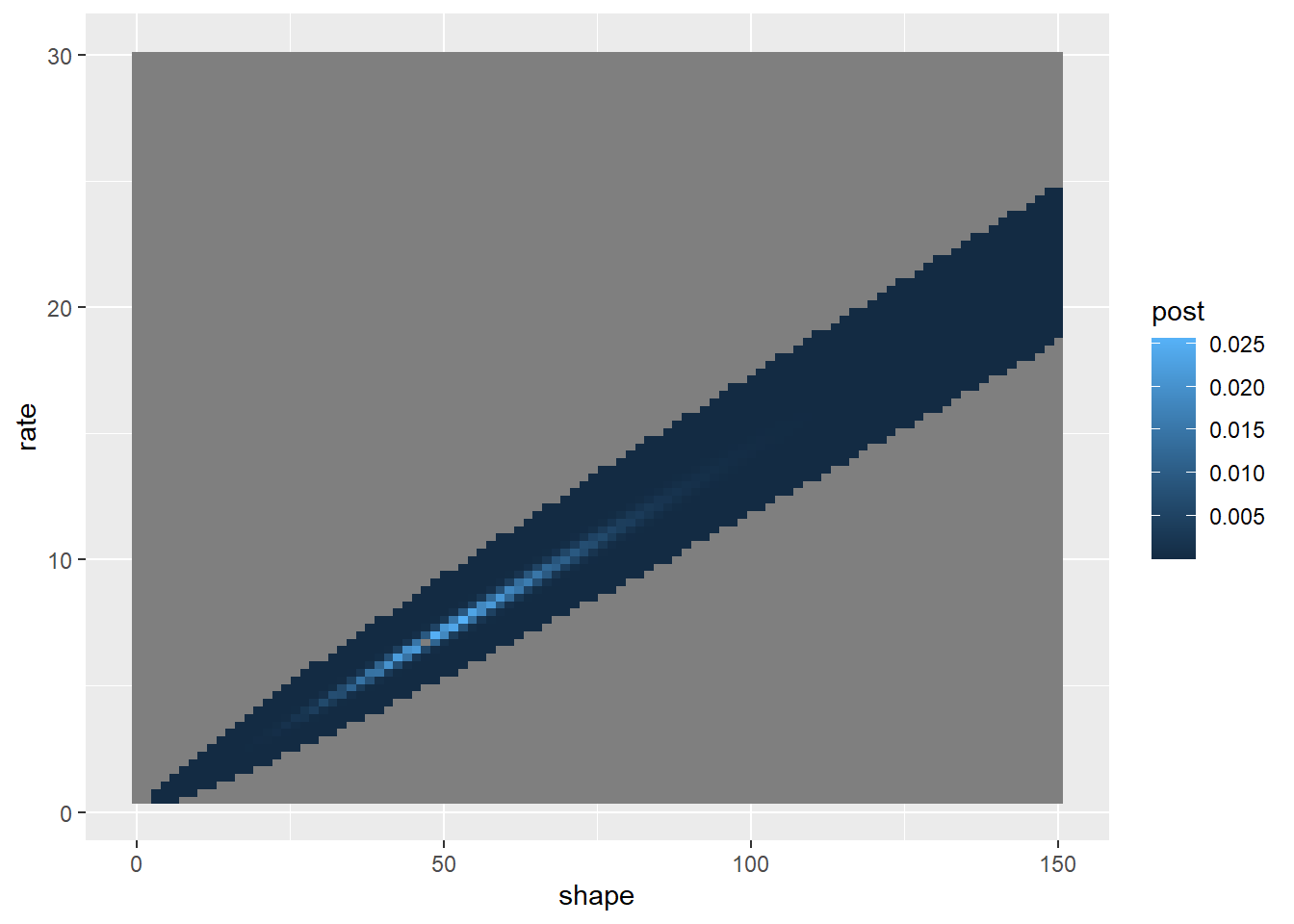And we are trying to fit a Gamma distribution to these data: ###### Error is modeled as gamma distributed hist(Myx$titer,freq=FALSE)
curve(dgamma(x,shape=40,scale=0.15),add=T,col="red")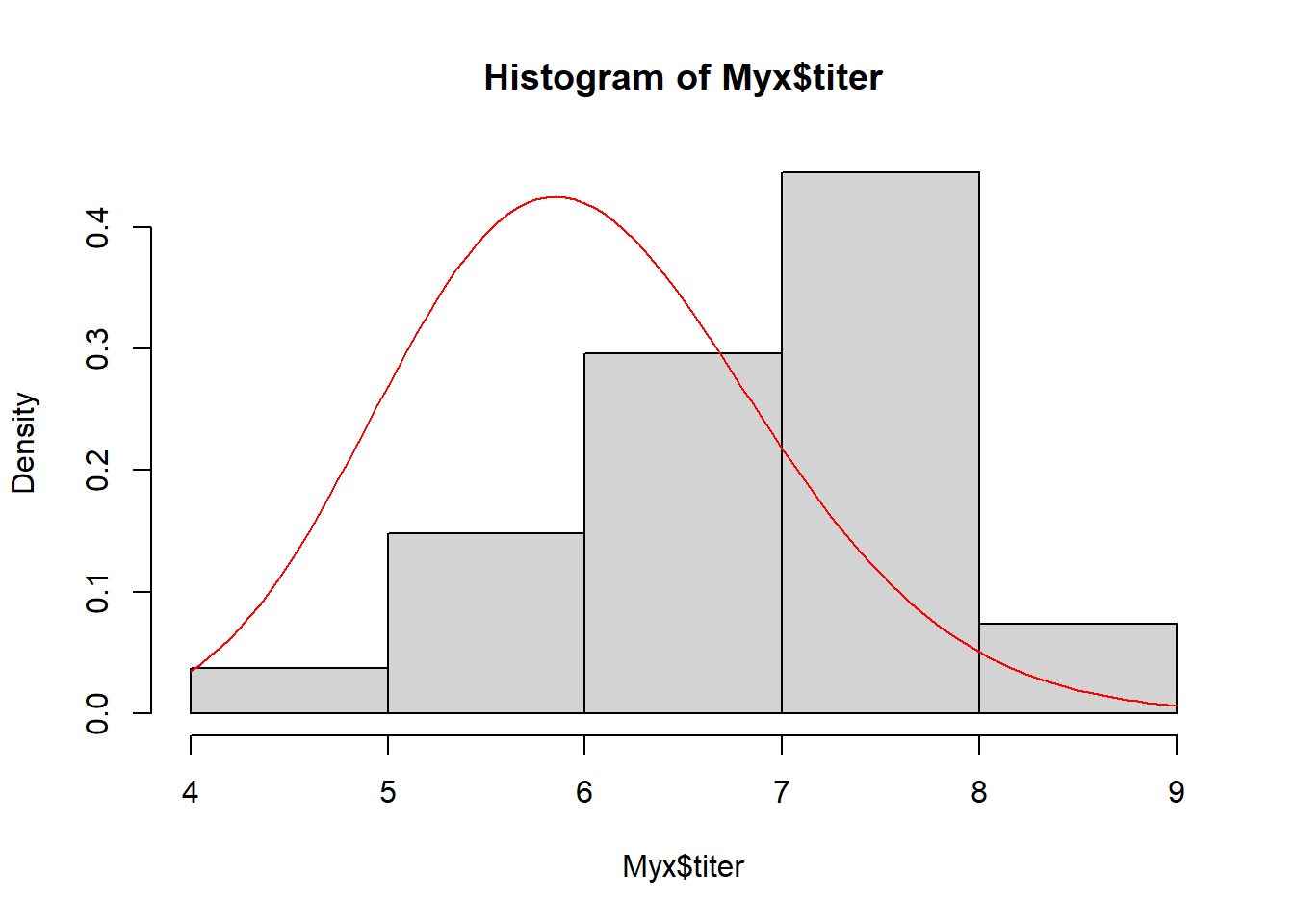We already have a likelihood function for this problem! Note that we are now looking at real likelihoods and not log likelihoods!

##########
# recall our likelihood function for these data (not on log scale this time!)

GammaLikelihoodFunction <- function(params){
prod(dgamma(Myx$titer,shape=params['shape'],scale=params['scale'])) } params <- c(40,0.15) names(params) <- c("shape","scale") params ## shape scale ## 40.00 0.15 GammaLikelihoodFunction(params) ##  2.906766e-22 And we recall that the 2D likelihood surface looks something like this (looks a bit different without the log transform though!): ############## # define 2-D parameter space (in real probability scale)! ############## shapevec <- seq(10,100,by=0.1) scalevec <- seq(0.01,0.3,by=0.001) ############## # define the likelihood surface across this grid within parameter space ############## likelihood2D <- matrix(nrow=length(shapevec),ncol=length(scalevec)) # initialize storage variable newparams <- params for(i in 1:length(shapevec)){ newparams['shape'] <- shapevec[i] for(j in 1:length(scalevec)){ newparams['scale'] <- scalevec[j] likelihood2D[i,j] <- GammaLikelihoodFunction(newparams) } } ############ # Visualize the likelihood surface ############ image(x=shapevec,y=scalevec,z=likelihood2D,zlim=c(1e-70,1e-17),col=topo.colors(12)) contour(x=shapevec,y=scalevec,z=likelihood2D,levels=c(1e-18,1e-17),add=T)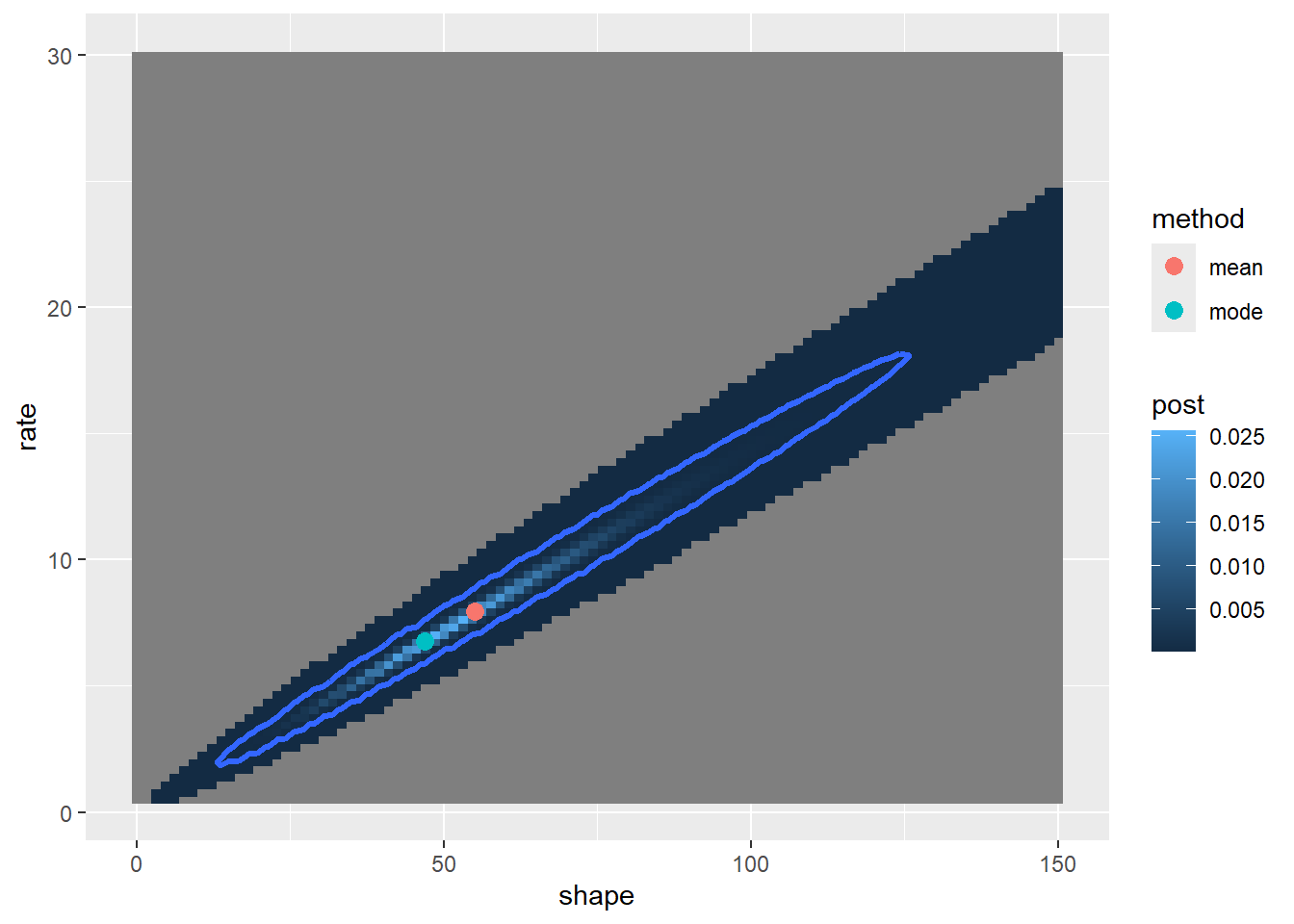Now let’s use this 2-D likelihood surface as a jumping-off point for a brute-force Bayesian solution to this problem! First we need to set priors for the shape and scale parameters… For example, we could set uniform distributions for these parameters. For this example, let’s imagine a prior in which all pixels in the above image are equally likely: ############# # compute the area of each pixel (for probability density computation) pixelArea <- 0.0001 # for determining probability densities ############## # define the prior probability surface across this grid within parameter space ############## prior2D <- matrix(1, nrow=length(shapevec),ncol=length(scalevec)) # initialize prior prior2D <- prior2D/length(prior2D) ############ # Visualize the 2-D prior distribution ############ image(x=shapevec,y=scalevec,z=prior2D,zlim=c(0,0.001),col=rainbow(10))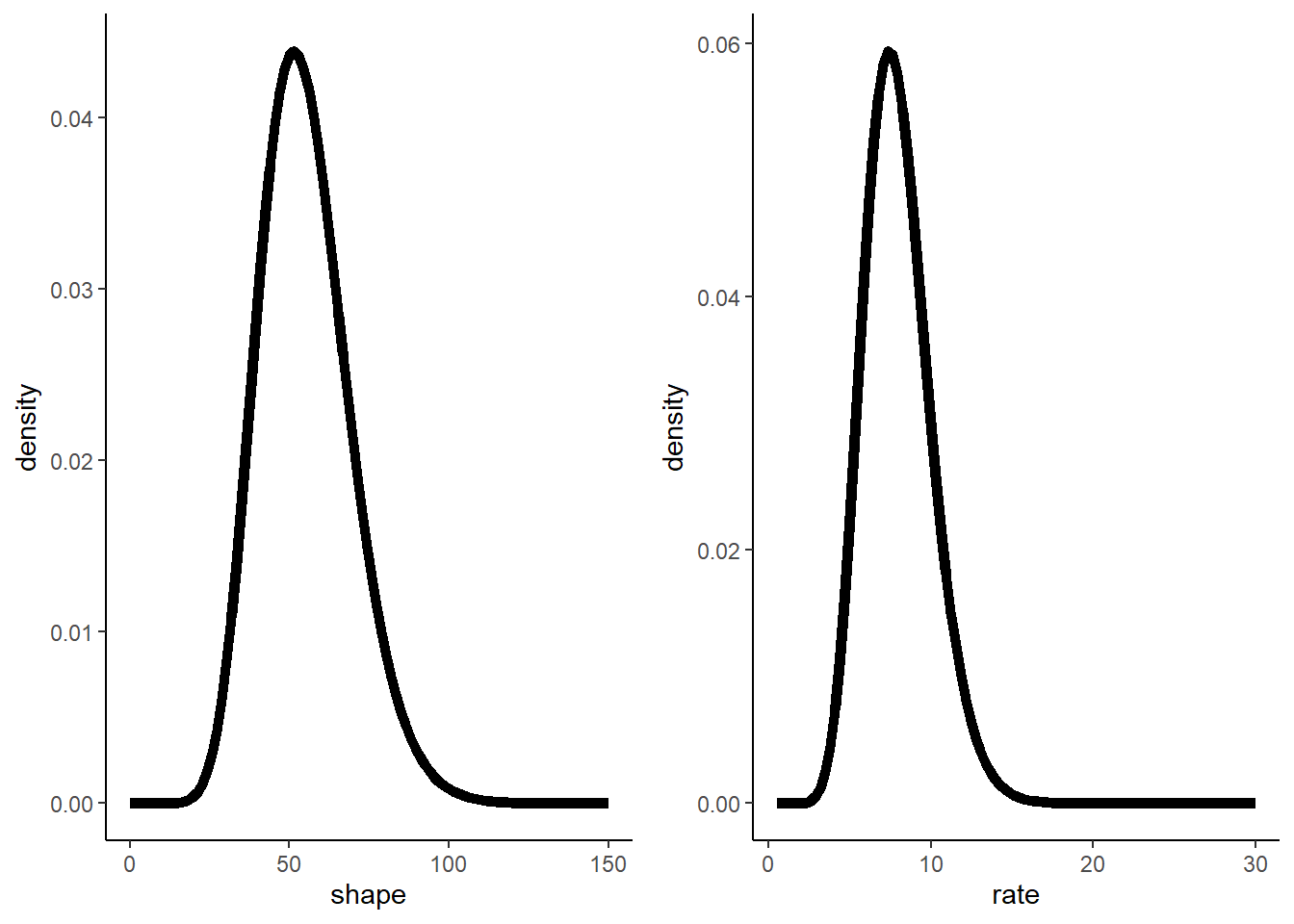Okay, not very interesting! But now we have the raw information we need to apply Bayes’ rule! ########### # Apply Bayes Rule! weighted.likelihood <- prior2D * likelihood2D # numerator of Bayes rule normalization.constant <- sum(weighted.likelihood) # denominator of Bayes rule posterior2D <- weighted.likelihood/normalization.constant ############ # Visualize the 2-D posterior distribution ############ image(x=shapevec,y=scalevec,z=(posterior2D/pixelArea),zlim=c(0,5),col=topo.colors(12)) contour(x=shapevec,y=scalevec,z=(posterior2D/pixelArea),levels=c(1:4),add=T,drawlabels=FALSE)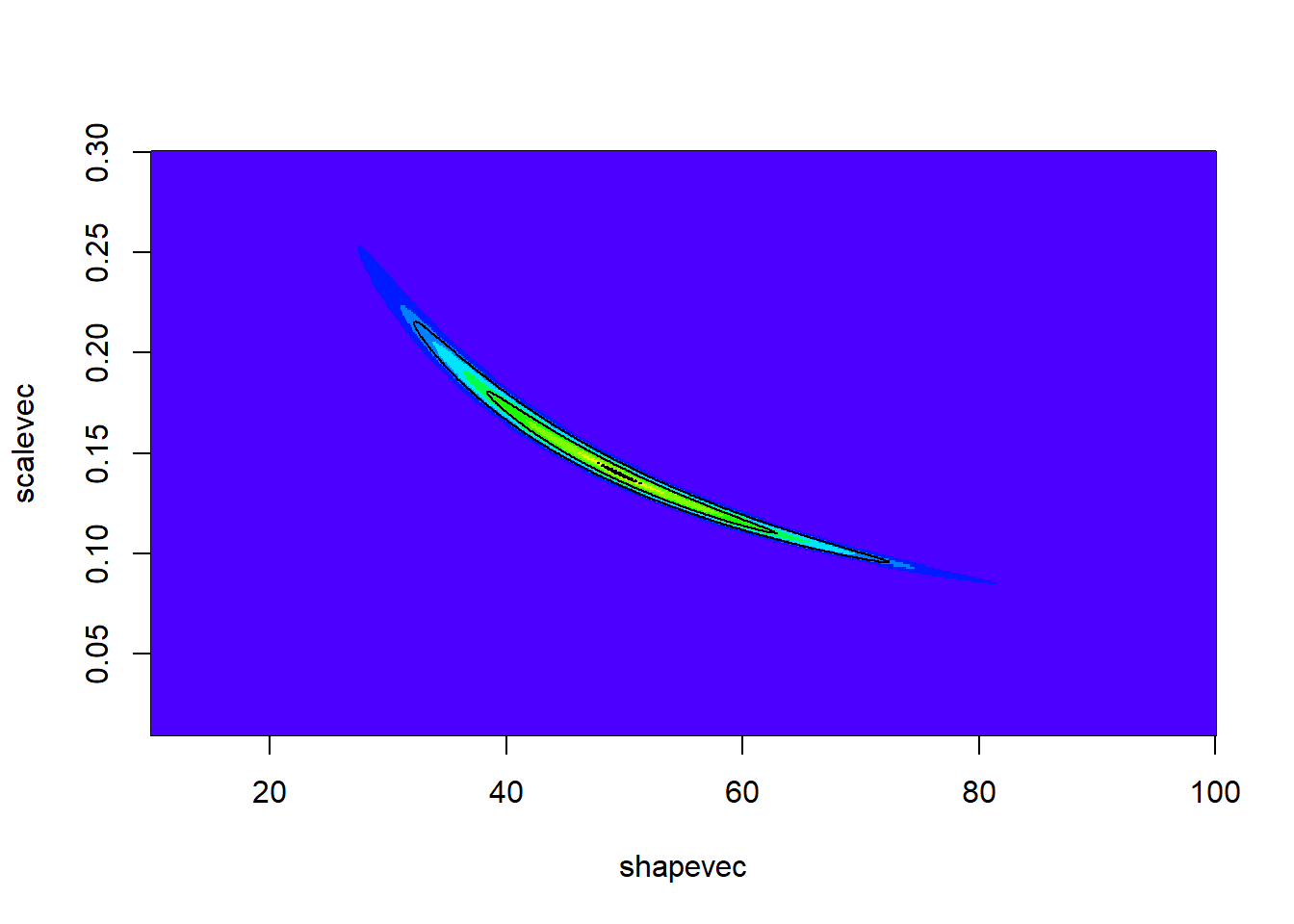Now we can use this posterior distribution to get our point estimates and parameter uncertainty estimates. We could just take the 2d posterior distribution surface and draw the contour containing 95% of the probability density: First let’s find the contour line below which only 5% of the probability density is contained (this represents an HPD credibal interval): ########### # try to find the contour that contains 95% of our degree of belief! possible.contours <- data.frame(contour = seq(0.13e-4,1e-4,length=100), quantile = NA) i=1 for(i in 1:nrow(possible.contours)){ ndx <- which(posterior2D<possible.contours$contour[i],arr.ind = T)
possible.contours\$quantile[i] <- sum(posterior2D[ndx])
}

head(possible.contours,10)
##         contour   quantile
## 1  1.300000e-05 0.03484278
## 2  1.387879e-05 0.03803099
## 3  1.475758e-05 0.04110702
## 4  1.563636e-05 0.04417352
## 5  1.651515e-05 0.04751733
## 6  1.739394e-05 0.05046922
## 7  1.827273e-05 0.05369474
## 8  1.915152e-05 0.05674380
## 9  2.003030e-05 0.05980036
## 10 2.090909e-05 0.06315809

From here we can see that the contour line at posterior probability 1.739394e-05 encloses 95% of the probability density

###########
# Visualize the 2D credible region (HPD)

q95 <- 1.739394e-05
image(x=shapevec,y=scalevec,z=posterior2D,zlim=c(0.5e-11,5e-4),col=topo.colors(12))
contour(x=shapevec,y=scalevec,z=posterior2D,levels=q95,add=T,lwd=3,col="red",drawlabels=FALSE)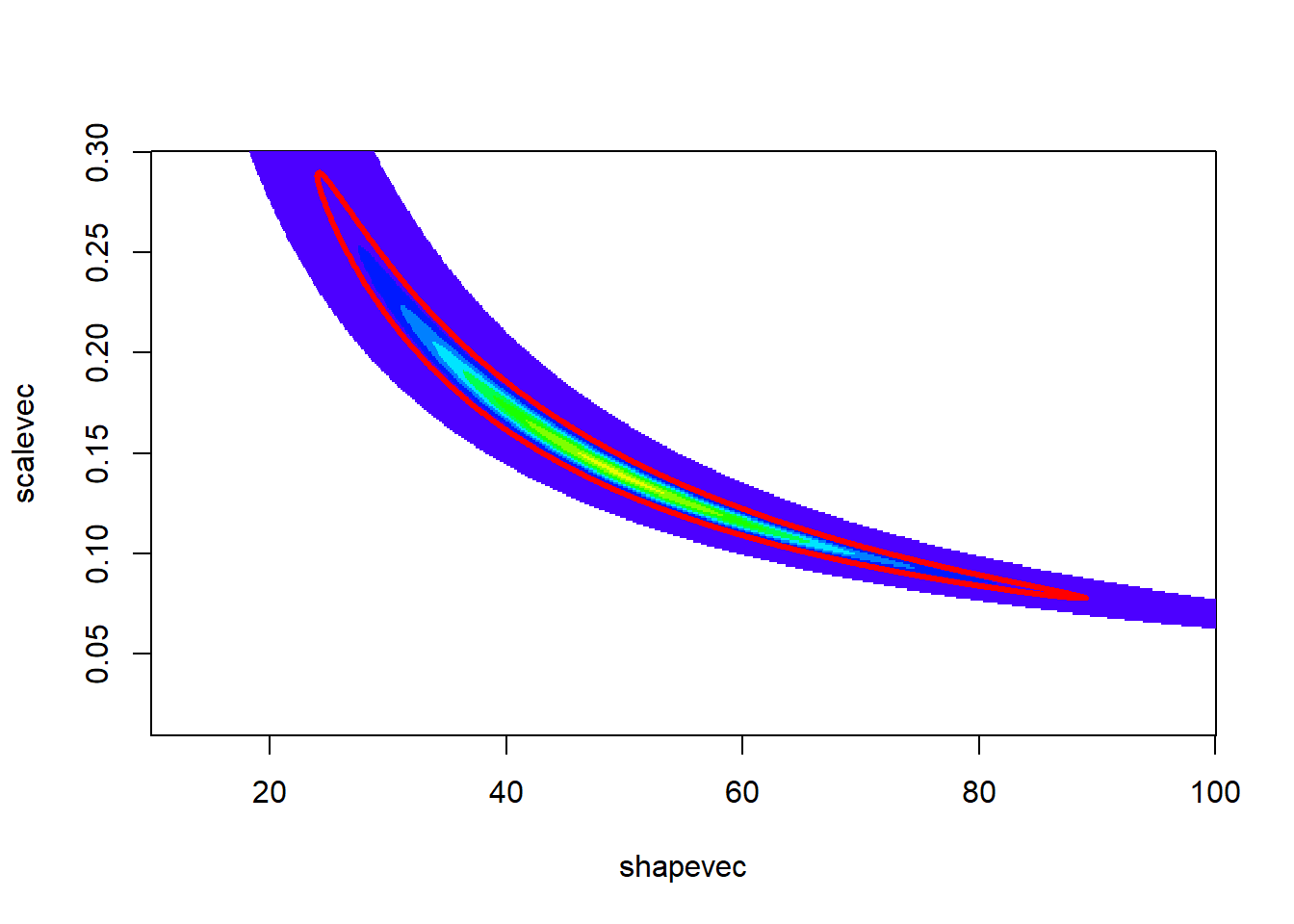Where is our point estimate? Let’s find the posterior mean and mode!

#############
# Visualize a point estimate

image(x=shapevec,y=scalevec,z=posterior2D,zlim=c(0.5e-11,5e-4),col=topo.colors(12))

meanshape <- sum(shapevec*posterior2D)
meanscale <- sum(scalevec*posterior2D)
points(meanshape,meanscale,pch=20,cex=2,col="red")  # posterior mean

mode <- which(posterior2D==max(posterior2D),arr.ind = T)
points(shapevec[mode],scalevec[mode], pch="X",col="black",cex=1.5)  # posterior mode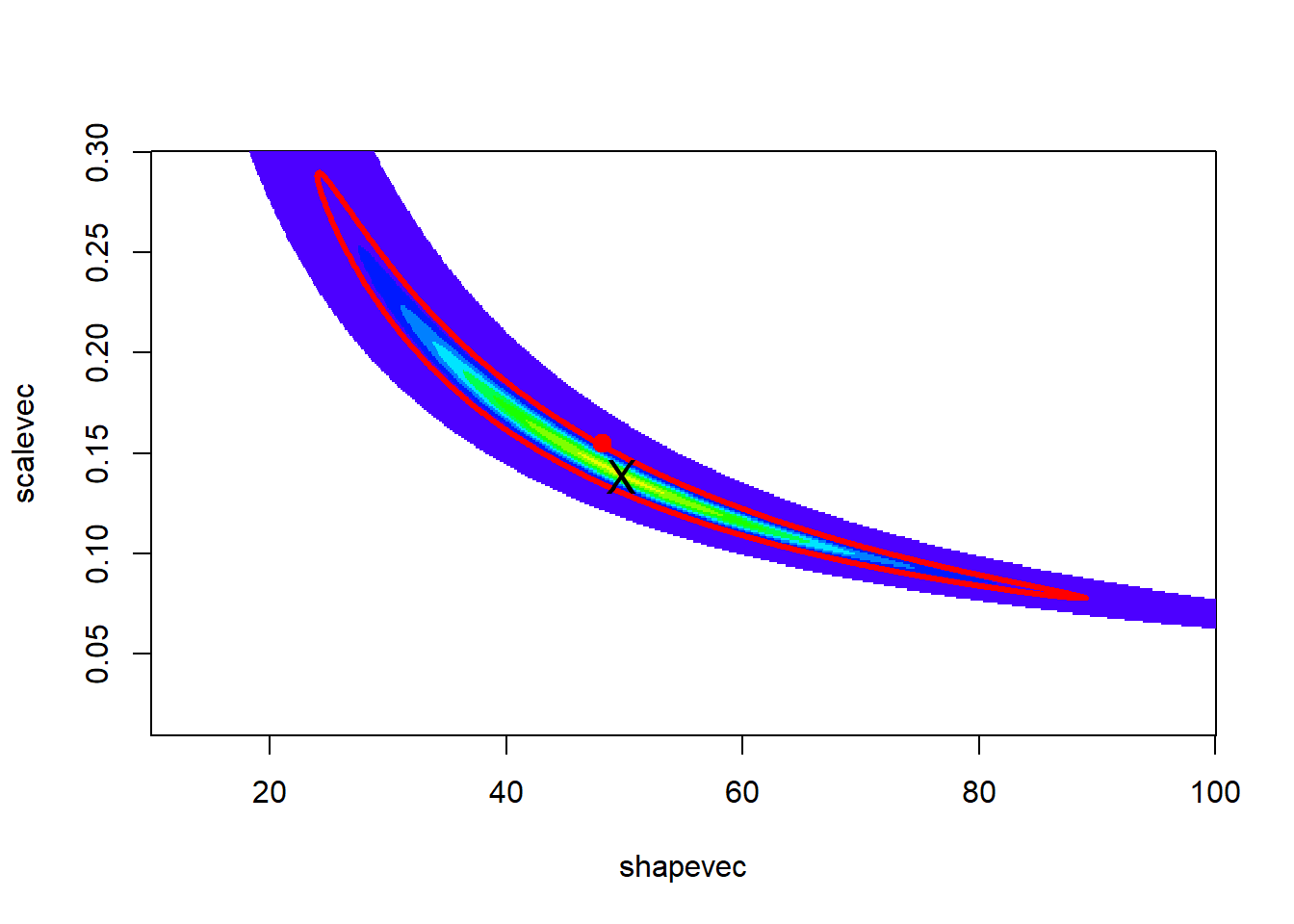We can also plot out the marginal posterior distributions for the parameters separately. For example, the probability that the ‘shape’ parameter falls within a given range, regardless of the other variable:

##########
# Plot out posterior distributions separately for each parameter

marginal.dist.shape <- apply(posterior2D,1,mean)     # factor out the scale param- focus only on the shape param.
plot(shapevec,(marginal.dist.shape/sum(marginal.dist.shape))/0.1,type="l",lwd=2,col="blue",ylab="probability density",main="Posterior probability")
abline(v=meanshape)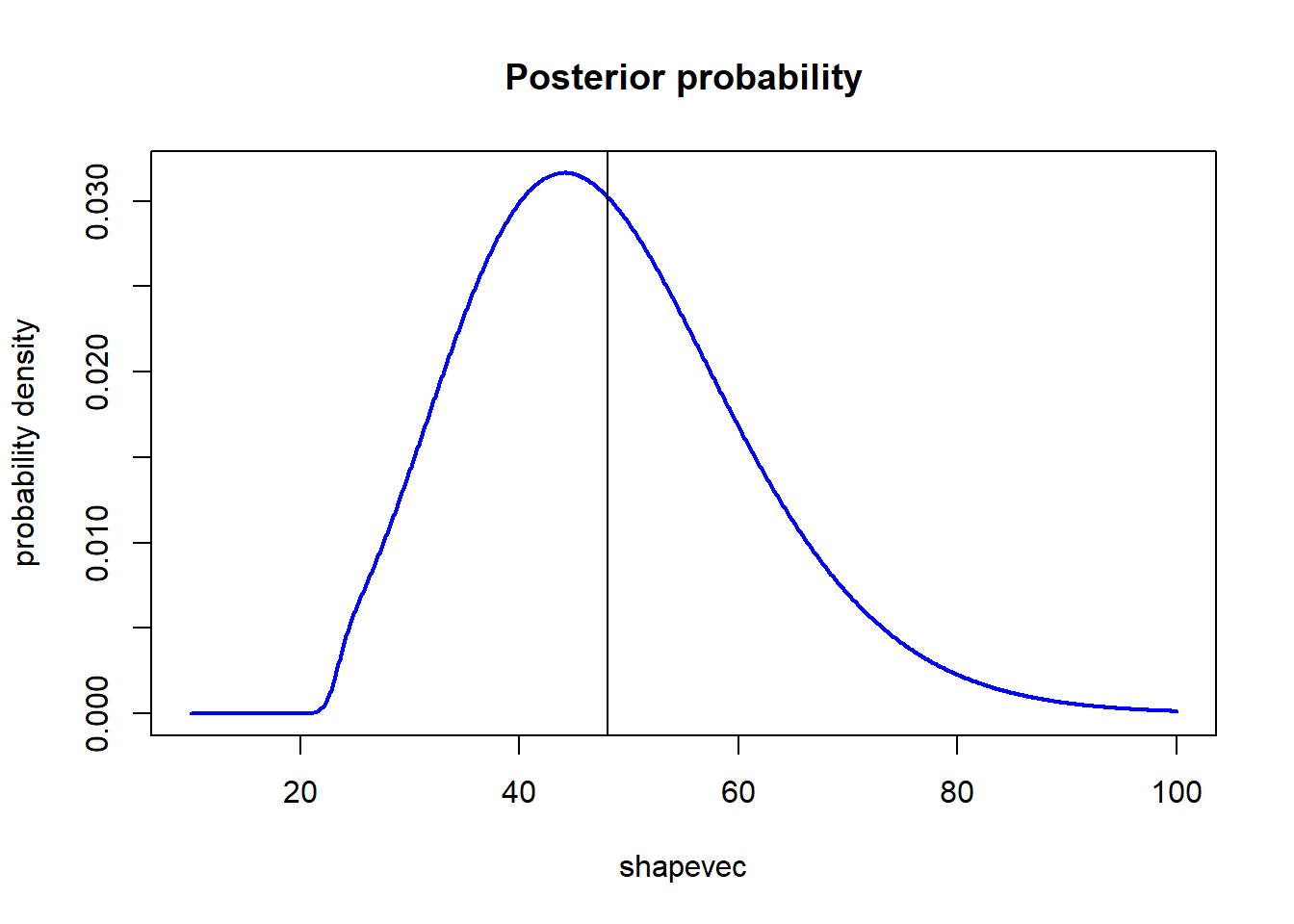marginal.dist.scale <- apply(posterior2D,2,mean)
plot(scalevec,(marginal.dist.scale/sum(marginal.dist.scale))/0.001,type="l",lwd=2,col="blue",ylab="probability density",main="Posterior probability")
abline(v=meanscale)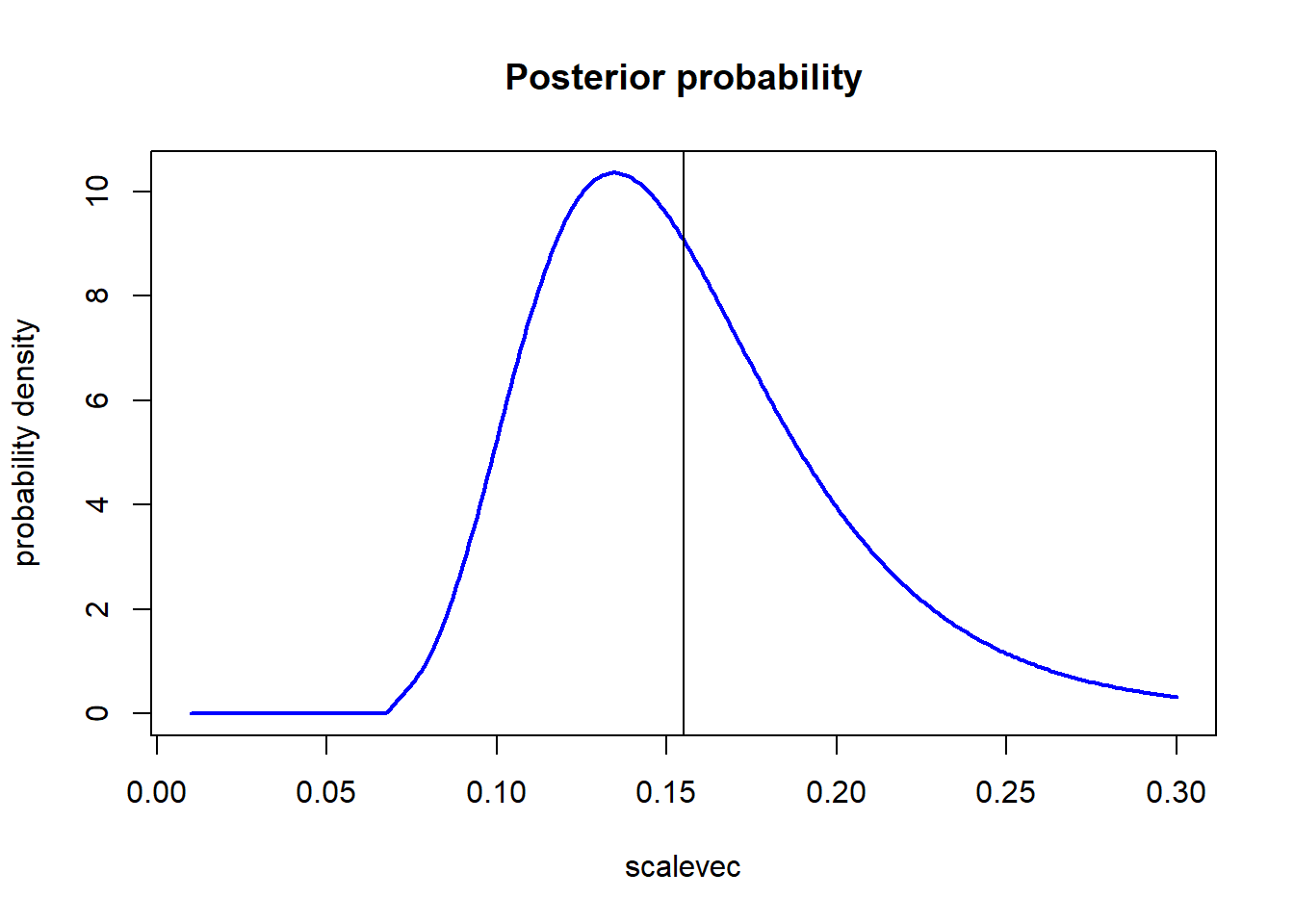meanshape
##  48.08924
meanscale
##  0.1549478

And from here we can compute the posterior mean and Bayesian credible intervals.

How do our Bayesian estimates compare with the maximum likelihood estimates?? (shape = 49.3666607 scale = 0.1402629)

We can also sample directly from this 2-D posterior distribution:

################
# Sample parameters from the joint posterior

SampleFromPosterior <- function(n){
shape <- rep(shapevec,times=length(scalevec))
scale <- rep(scalevec,each=length(shapevec))
jointparams <- data.frame(shape=shape,scale=scale)
probs <- as.vector(posterior2D)
samples <- sample(c(1:length(probs)),size=n,replace=TRUE,prob=probs)
jointparams[samples,]
}

samples<-SampleFromPosterior(n=10000)
par(mfrow=c(3,2))
plot(samples,col=1:10000)
plot(samples,type="l")
plot(ts(samples[,1]),xlab="sample",ylab="shape")
plot(ts(samples[,2]),xlab="sample",ylab="scale")
hist(samples[,1],40,xlab="shape",main="histogram of shape param")
hist(samples[,2],40,xlab="scale",main="histogram of scale param")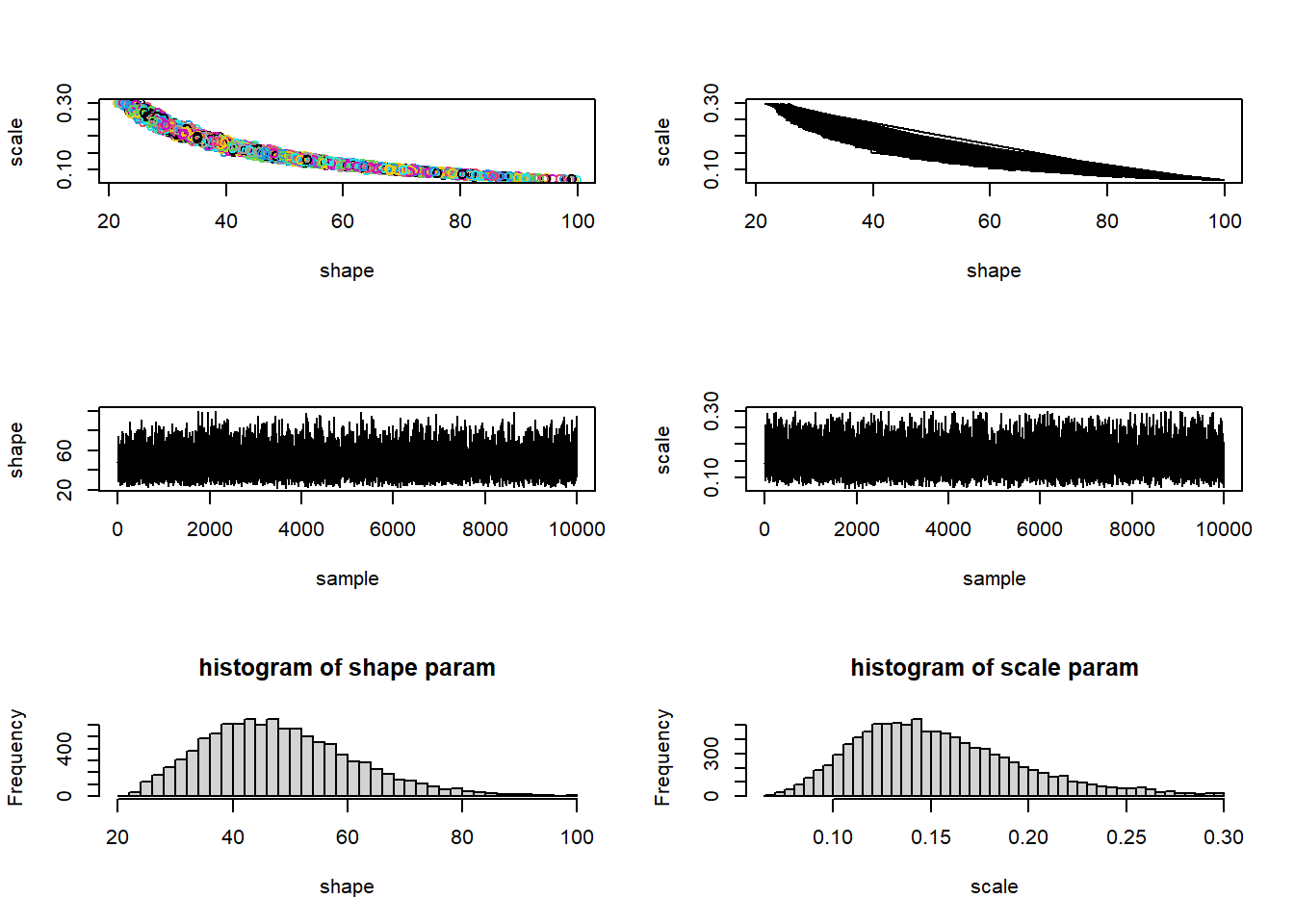par(mfrow=c(1,1))

### Markov Chain Monte Carlo

Now in many cases, we simply won’t have the computational power to partition our parameter space into discrete pixels and completely evaluate the posterior probability for all n-dimensional pixels in that space. In these cases, we tend to harness ingenious algorithms known as Markov-Chain Monte Carlo (MCMC). This is the focus of the next lecture.

–go to next lecture–